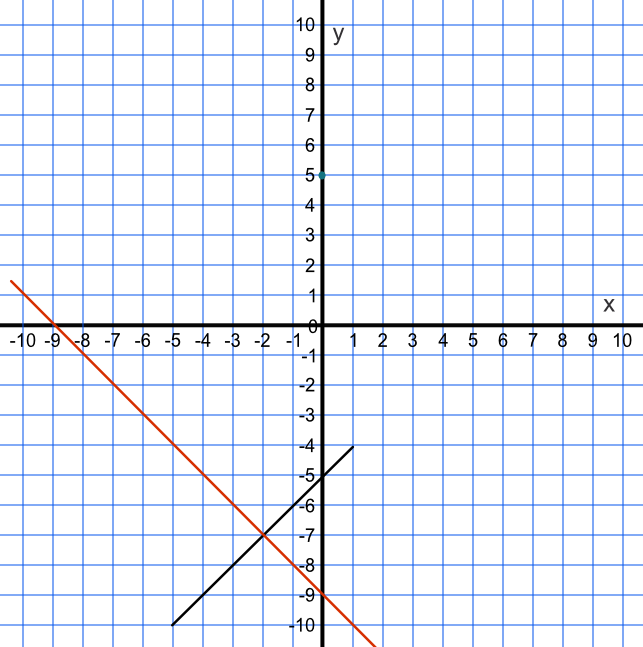Midpoint of a Line Segment

## Midpoint of a Line Segment

The midpoint of a line segment is the halfway point between the two ends of a line. You can work it out by adding the two x-coordinates together and dividing by two to get the x-coordinate of the midpoint; and then repeat with the y-coordinate.

The formula for the midpoint of a line segment is (frac(x_1+x_2)(2), frac(y_1 + y_2)(2)).

## Example 1

What is the midpoint between (3,11) and (7,15)?

Add the two x-coordinates then divide by two = frac(3 + 7)(2) = 5

Add the two y-coordinates then divide by two = frac(11 + 15)(2) = 13

Midpoint coordinate is (5, 13)

## Example 2

A line segment is drawn from (-5,-10) to (1,-4). A perpendicular line passes through the midpoint of this line segment.

What is the value of the y-coordinate on the perpendicular line when x = 0?

Work out the equation of the line segment as y=mx+x

gradient = frac(6)(6) = 1

For the point (-5, -10)

-10 = 1 xx -5 + c

c = -5

Check with other point: -4 = 1 x 1 - 5 ✔

Line segment has equation y=x - 5

Midpoint of line segment: x=frac(-5 + 1)(2) = -2

And y = frac(-10 + -4)(2) = -7

Midpoint is therefore (-2, -7)

The perpendicular line has a gradient of frac(-1)(m) = frac(-1)(1) = -1

Perpendicular line passes through the point (-2, -7) and substituting

-7 = -1 x -2 + c gives c=-9

And the equation of the perpendicular line is y = -x - 9

When x = 0, y = 0 - 9